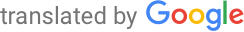Machine-translated page for increased accessibility for English questioners.

# Artificial intelligence and natural language processing

1. Database. Data storage, addressing of records. Indexing and hashing for multiple attributes, bitmap indexes, dynamic hashing. Query evaluation, transformation rules, statistics and estimates. Query and schema optimization. Transaction processing, outages and recovery. Similarity search.
`PA152, PA128`
2. Computational logic. Resolution method in predicate logic. Refinement of the resolution and the Horn clause. SLD resolution. Computational model of a logic program. Prolog language. Tabular proofs in propositional, predicate and modal logic. Inductive inferences in propositional and predicate logic. Multivalued logics.
`IA008`
3. Complexity and algorithms for difficult problems. Complexity classes, reductions, complete problems. Basic temporal and spatial complexity classes, the relationship between determinism and nondeterminism. P vs NP problem. Approximate algorithms. Use of the linear programming problem for the construction of approximate algorithms. Random algorithms. Heuristic approaches.
`IA012, IA101`
4. Statistics. Descriptive statistics, functional and numerical characteristics of characters. Discrete and continuous random variables (NV), basic distribution. Numerical characteristics of NV. Central limit theorem. Point estimates, confidence intervals, testing of statistical hypotheses, level of significance. Linear regression, total F-test, partial t-tests. Statistical methods and evaluation of experiments.
`MV011, MA012`
5. Games, modeling, simulation. Games and basic game strategies. Modeling, types of models, computer models, simulations. Markov models. Feedback, balance. Agent modeling, network modeling. Modeling of thinking, learning, evolution. Cellular automata, production systems.
`IV109, IV111, PA154, M7190`
6. Soft Computing Methods. Neural networks. Genetic algorithms. Complex systems, natural complex systems, social insects. Iterative local search, simulated annealing, search tab.
`PV021, IV109, IA101, IV126`
7. Constraint searching and programming. Solve problems by searching the state space. Consistency and algorithms for binary and non-binary conditions. Tree search without / with consistency techniques. Constraint modeling, global conditions.
`PA163`
8. Machine learning and knowledge acquisition. Classification, clustering, search for common patterns and association rules. Multirelational learning. Data preprocessing. Mining from text and web documents. Results validation methods.
`PV056, PA164, PA055`
9. Scheduling and planning. Graham's classification. Scheduling using control rules, constraints, local search, and mathematical programming. Project planning, task planning.
`PA167`
10. Natural language processing. Automatic morphological analysis. Recognition and generation of sentence structure, grammar, basic types of parsing. Semantic analysis of sentences, logical analysis of natural language. Pragmatic level, communication situation. Corpora, statistical and rule marking.
`PA153, IV029, IA161, PA164, PA154`
11. Methods of representation and derivation of knowledge. Representation of knowledge using rules, frameworks, semantic networks. Deductive and inductive derivation. Forward and backward chaining of rules. Derivation with uncertainty.
`PA153, IA008`